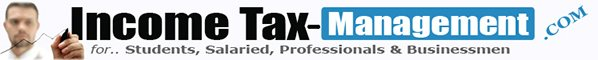# Manner of Computation of Interest payable by the Tax Payer under the Income-tax Act. [Rule 119A]

Under the Income-tax Act, different types of interests are levied for various kinds of delays/defaults. In this part, you can gain knowledge about the provisions of section 234A, 234B and 234C dealing with interest levied for (i) delay in filing the return of income; (ii) non-payment or short payment of advance tax; and (iii) non-payment or  short payment of individual instalment or instalments of advance tax (i.e., deferment of advance tax).

Before understanding the provisions of section 234A, 234B and 234C it is important to understand the provisions of Rule 119A which gives the manner of computation of interest under the Income-tax Act.

As per Rule 119A, while calculating the interest payable by the taxpayer or the interest payable by the Central Government to the taxpayer under any provision of the Act, the following rule shall be followed :

a)         where interest is to be calculated on annual basis, the period for which such interest is to be calculated shall be rounded off to a whole month or months. For this purpose any fraction of a month shall be ignored and the period so rounded off shall be deemed to be the period in respect of which the interest is to be calculated;

b)         where the interest is to be calculated for every month or part of a month comprised in a period, any fraction of a month shall be deemed to be a full month and the interest shall be so calculated;

c)         the amount of tax, penalty or other sum in respect of which such interest is to be calculated shall be rounded off to the nearest multiple of one hundred rupees. For this purpose, any fraction of one hundred rupees shall be ignored and the amount so rounded off shall be deemed to be the amount in respect of which the interest is to be calculated.

E.g. If we want to compute interest under section 234A on Rs. 8,489 for 3 months and 10 days, then as per Rule 119A discussed above, while computing the amount liable to interest, any fraction of Rs. 100 is to be ignored and, hence, we will ignore Rs. 89 and the balance amount will come to Rs. 8,400. Interest will be computed on Rs. 8,400. Further, the period of 10 days will be considered as full month and, hence, interest will be computed for 4 months.

### You may also like ...